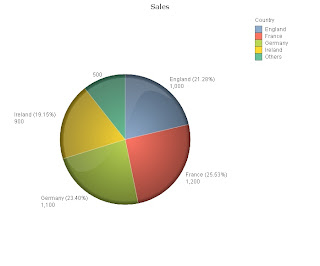## Wednesday, 23 June 2010

### More on Dual - "Others" in chart using AGGR

In a previous blog post (More On Dual - http://qliktips.blogspot.com/2009/09/more-on-dual.html) I proposed a method for getting better labels in a Pie chart by using Dual to create the label and the value in the expression:

Dual(Country & ' (' & Num(Sum(Sales)/Sum(Total Sales),'0.00%') & ')' & chr(13) & Num(Sum(Sales),'#,##0'), Sum(Sales))

A problem has been pointed out to me by one of my readers - if the number of slices is restricted (10 by default), then the "Others" does not display correctly:We can fix this by limiting the number of dimensions using an AGGR statement with RANK in a calculated dimension:

=If(aggr(rank(Sum(Sales)), Country) <= 4, Country, 'Others')

So here, I am checking if the rank of the dimension is less than or equal to 4. If so, just use the Country name, if not, use "Others".

Now, there is still a slight problem with this and the Dual - the name is still blank. So, we need to modify the expression to check for a blank name:

Dual(If(Len(Country)>0,Country,'Others') & ' (' & Num(Sum(Sales)/Sum(Total Sales),'0.00%') & ')' & chr(13) & Num(Sum(Sales),'#,##0'), Sum(Sales))

(It will be blank because there are actually multiple values).

So, all should be well!

Of course, this doesn't just apply to Pie charts (thankfully!) - this method of restricting the dimension using Rank and AGGR can be used in any chart - including Straight Tables!